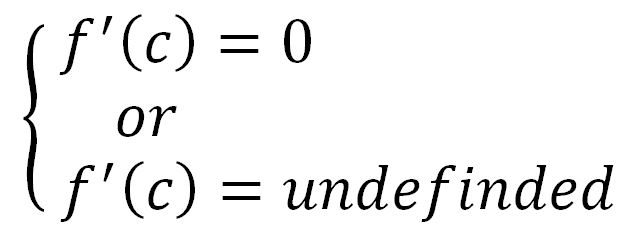# Critical number & maximum and minimum values#### All You Need in One Place

Everything you need for better marks in primary, GCSE, and A-level classes.#### Learn with Confidence

We’ve mastered the UK’s national curriculum so you can study with confidence.#### Instant and Unlimited Help

0/3
##### Intros
###### Lessons
1. How to describe graphs of functions?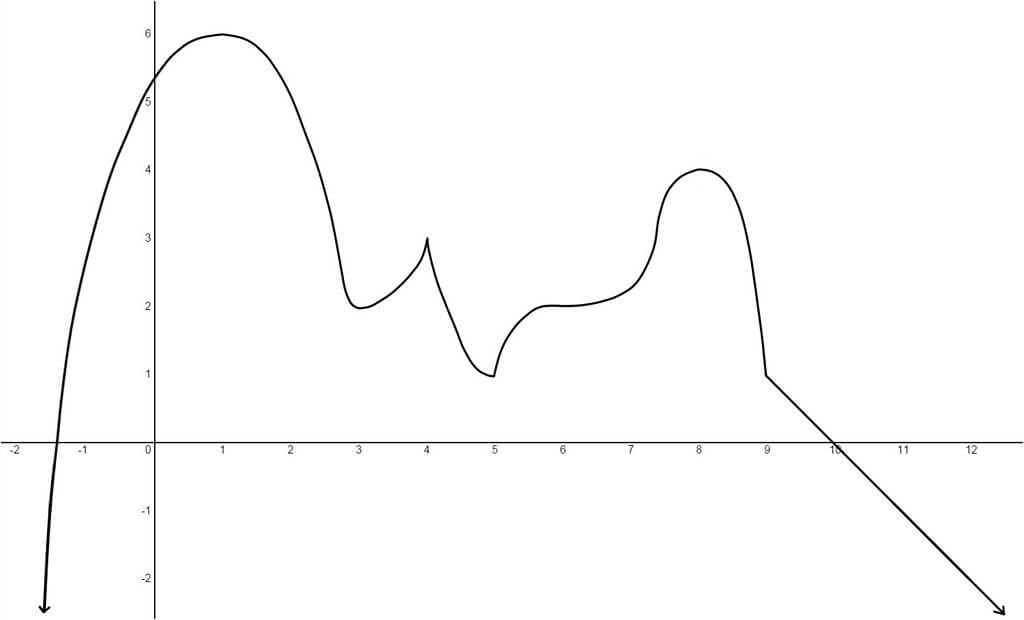2. $\bullet$ local maximum
$\bullet$ local minimum
$\bullet$ critical number

3. state the:
$\bullet$ absolute maximum
$\bullet$ absolute minimum

4. on the interval,
$-1\leq x\leq 12\,$state the:
$\bullet$ absolute maximum
$\bullet$ absolute minimum
0/10
##### Examples
###### Lessons
1. Find the critical numbers of the function:

1. $f(x)={^3}\sqrt{x^{2}-5x}$

2. $f(x)=x^{\frac{1}{3}}-x^{-\frac{2}{3}}$
2. First Derivative Test: a test to determine whether or not$\ f$ has a local maximum or minimum at a critical number
 First Derivative Test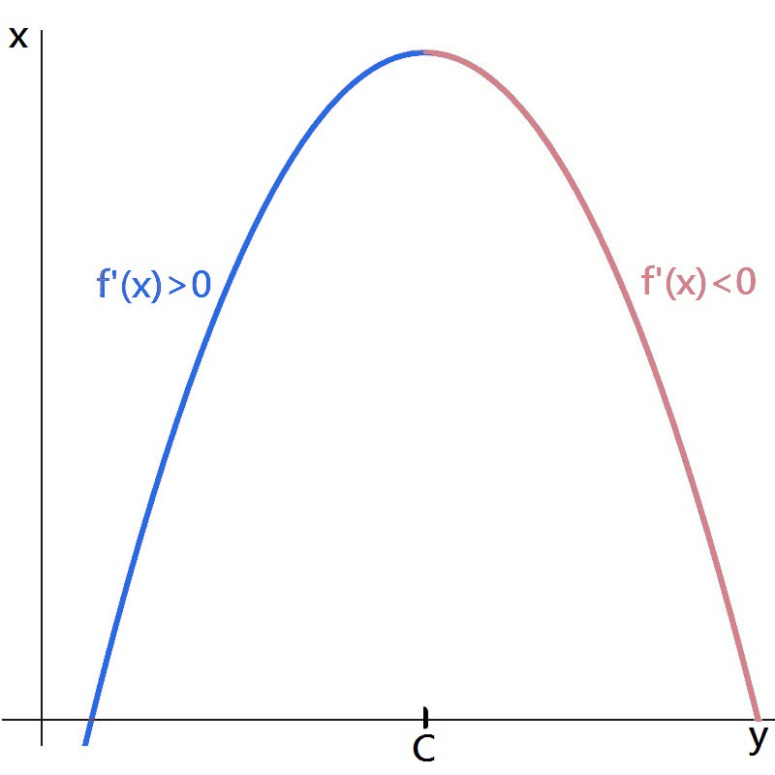local maximum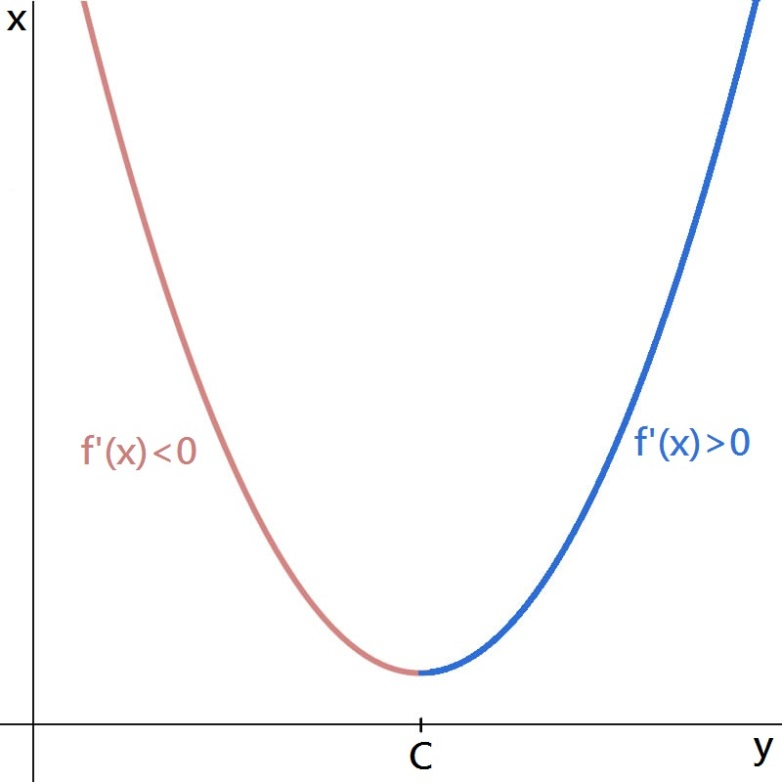local minimum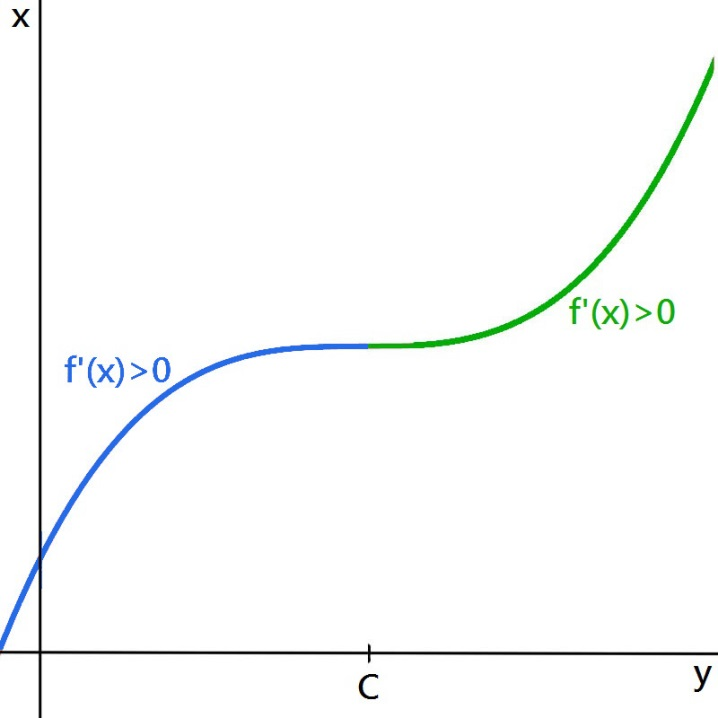no maximum or minimum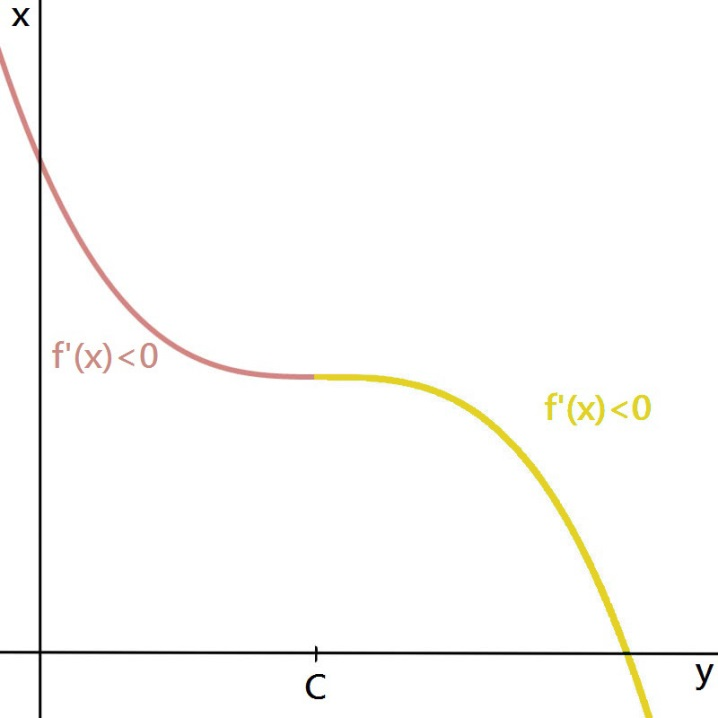no maximum or minimum
1. $f(x)=3x^{5}-15x^{4}+25x^{3}-15x^{2}+5$
1. Find the critical numbers.
2. On what intervals is $f$ increasing or decreasing?
3. Find the local maximum and minimum values.
4. Sketch the graph.
5. Find the absolute maximum and minimum values.
2. The Closed Interval Method
To find the absolute maximum and minimum values of a continuous function $f$ on a closed interval [a, b]:
1.Find the values of $f$ at the critical numbers of $f$ in (a, b).
2.Find the values of $f$ at the left-endpoint and right-endpoint of the interval
3.Compare all values from steps 1 and 2: the largest$\$is the absolute maximum value; the smallest$\$is the absolute minimum value.
1. Find the absolute maximum and minimum values of the function:

$f(x)=3x^{5}-15x^{4}+25x^3-15x^2+5$
$-\frac{1}{2}\leq x\leq\frac{1}{2}$
###### Topic Notes
Another powerful usage of differential calculus is optimization, for example, finding the number of products needed to be sold at a store to maximize its monthly revenue or to minimize its monthly costs. In this section, we will link the application of differential calculus with finding the local extrema, the maxima and minima, of a function.

critical number: a number$\ c$ in the domain of a function$\ f$ such that: